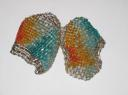# Introduction

One of my favorite things about MATLAB is that I can write a mathematical algorithm using the same notation appearing in a technical book or paper. For algorithms that are naturally matrix-based, the economy and clarity of the MATLAB code contribute to the program's elegance.

What constitutes good code? Here are some possible characteristics:

• fast
• clear
• maintainable
• efficient
• short
• memory-efficient
• "clever" – for example, see the MATLAB golf contest
• reliable

During my first year at MathWorks, 1987, a customer contacted us that he was having trouble translating and debugging an algorithm. The algorithm the customer was interested in was for solving a least-squares problem with constraints that the coefficients be non-negative. He based his MATLAB implementation on the Fortran code supplied in Lawson and Hansen's wonderful book, Solving Least Squares Problems (Prentice-Hall, 1974.). The Fortran code and the translated M-file were equivalent in length, in the range of 300 lines of code.

Taking a look at the code, I decided that though I had used the Fortran routine before, it was now time to dig in and understand the algorithm more. For that I turned to the relevant chapter where I found some pseudo-code. Using the pseudo-code as the basis for coding the algorithm, the resulting M-file was about 80 lines long. The algorithm originally shipped in MATLAB as the function nnls, and has since be renamed to lsqnonneg.

You won't always see the algorithm you want to code expressed in the language of linear algebra even when it might be appropriate. Part of the art of using MATLAB well is learning when and how to cast algorithms into the linear algebra framework. There are often several good ways to solve such a problem, with competing solutions having different benefits. Recognizing which code characteristics are important in any given situation contributes to the art. There is invariably room for esthetics when programming in MATLAB.These earrings, which I found shortly before the first MATLAB User Conference in 1993, remind me of the beauty and flexibility of mathematics, linear algebra, and MATLAB.

This blog will discuss topics relevant to programming in MATLAB. My advice is to write readable, elegant code. One of our goals at MathWorks is to make sure that such code executes well and we continue to invest in MATLAB to ensure that this is so.

|